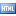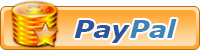• ###Contribution

Please support MyCockpit by considering contributing.Donate Here

• ###Staff

MyCockpit, Inc. Executive and Corporate Staff

Matt Olieman,

COO and Executive Vice-President

David Crist (Deesystems),

Larry Vandivier,

Co-Founder

MyCockpit, Inc. Support Staff

Forum Moderators

Geremy Britton

Forum Managers

Alan Dyer, FS2Phidgets

Gwyn Perrett (Westozy), Westozy's Mechanical Engineering

Nico Kaan, Leekseecon

Roberto Soriano (Dodiano), Dodiano's Airbus Forum

• ###Contact Information

MyCockpit Contact Information

News Releases

Editors@mycockpit.org

applications@mycockpit.org

Abuse

abuse@mycockpit.org

• # How to estimate/verify 737 Vspeeds

Tutorial Presented by Member xtreme3d

Some info on how to verify or estimate your Vs. This is out of a website I found a while ago. Really useful info for 737 Capts out there. No excuses now. Enjoy!!!

V-speeds

I use the following methods to calculate the V-speeds.

First very simple ones.
1. For 737-700 V2 is approximately take-off weight - 25.
Eg: For 60t, 60-25=35. V2 is 135kts.

2. For 737-800 V2 is approximately take-off weight - 20.

3. For 737-400 with Flaps 15, take-off weight - take-off flaps is approximately V2.

WEIGHT. //// V2 /// QRH V2 (Col.A)

65 (65-15) / 150 / 152

60 (60-15) / 145 / 146

55 (55-15) / 140 / 141

50 (50-15) / 135 / 135

45 (45-15) / 130 / 128

40 (40-15) / 125 / 122

As you can see our reference weight is 50t where the speed is exactly the same as QRH. Error above or below this weight is hardly 2kts which is quite reasonable. To be more accurate we can always add 2 kts to be on the safer side when weights are more than 50t. and subtract 2 kts when weights are less than 50t.

So far so good. The calculation of V speeds for Flaps 5 is slightly more lengthy.

The first step is same as in flaps 15, (take-off weight - take-off flaps). For flaps 5 the ref. weight. is 60 t. Clearly there won't be any error at this ref. weight and no need for any correction.

V2 for 60t with flaps 5 is: 60-5= 55 ie 155.

The error away from ref weight increases at a given rate. Suppose the weight is higher than ref weight. 60t. than we add one more flap that is our first flap.
V2 for 65t: 65-5=60+1=61 ie 161. Same as QRH.

Similarly if the weight is lower than 60t we subtract one more flap that is again flap one. V2 for 55t: 55-5=50-1=49 ie 149. Same as QRH.

Next printed weight in QRH is 50t which is 2 steps away from ref weight. So we also need to put 2 step correction. Interestingly, it is very easy. The second step of the correction is next flap.
V2 for 50t: 50-5=45-(1+2)=42 ie 142. Same as QRH.

Next weight is 45t. For this we jump to next flap for the correction.
V2 for 45t: 45-5=40-5=35 ie 135 which is only 1 kt less than QRH V2.

Next weight is 40t. For this we maintain the same correction as if we can not cross our take-off flaps. V2 for 40t: 40-5=35-5=30 ie 130. Same as QRH.

Now when we know the V2 for a set of weights, V2 for different weights can be found using interpolation.

For example: Actual take-off weight. is 53.5t

As we can find out V2 for 50t and 55t and that is 142 and 149. Now find the increase in speed for 3.5 t over 50t. Diff in V2 is 7kts/5t. Double this to get 1.4t/1t. 1.4x3=4.2+.7=4.9kts. V2 for 53.5t will be 142+5=147kts.

To get V1 we can remember a certain table.
Starting with 65t the diff between V1 and V2 is 10kts,
60t 11kts,
55t 12kts,
50t 13kts,
45t 15kts,
40t 17kts.
So V1 for 53.5t is 147-13=134kts.

The diff between V1 and Vr is just 2 kts up to landing weight of 55t. Above landing weight this diff is 4kts.

Vr for 53.5t is 134+2=136.

(For a rough estimate V1 is about 10 kts less than V2 and Vr is about 2 kts more than V1 generally.)

V Refs

Ref. Weight. is 40t. and a standard correction of minus 1 up to max landing weight. Above max landing weight it is 3kts.

Vref for 40t for flaps 30 is 40 x 3 = 120 -1 = 119kts.
" " " " " 40 is Vref 30 - 3 = 116kts.

Next just half the difference between Actual and ref weight. and then add back to ref weight. x 3 = V - 1 = Vref for that weight.

Example: Actual weight. 50t which is 10t more than ref weight.
10/2 = 5 + 40 = 45 x 3 = 135 -1 = 134. Same as QRH/FMC.

Vref for flaps 40 for 50t is Vref 30 - 4 = 130kts.
(Diff between Vref 30 and 40 is 3kts below 50t. 50t and above, it is 4kts.)
(Diff between Vref 30 and Vref 15 is about 15 kts.)

The above rule of thumb has no error and it is as good as your QRH speeds for flaps 30 and 40 up to landing weight of 55t. Above this weight standard correction of 1 kts is increased to 3kts)

To make the above calculation easy, like John's method we need to remember only ref speed of 120 kts. Just half the difference of weight between actual and the ref and multiply by 3. Add this to 120 and we have Vref + 1 kts for flaps 30.

Example: Actual landing weight is 50t. so difference is 10t. Half of that is 5. 5x3 = 15. 120+15 = 135 - 1 = 134kts. I leave to the pilot whether he would like to remember 119 or 120 as the reference speed for the convenience.

Another example: Act land weight is 49.4t diff is 9.4. Half of that is 4.7. It is then multiplied by 3. (4x3=12)+(.7x3=2.1) = 14.1 + 119 = 133kts.

I hope the above method will help pilots flying 737 to be within safe limits of operating speeds.

All the information can be found here:

http://www.b737.org.uk/vspeedcalc2.htm